## Table of ContentOpen Access

ARTICLE

# Coordinated Rotor-Side Control Strategy for Doubly-Fed Wind Turbine under Symmetrical and Asymmetrical Grid Faults

Quanchun Yan1,2,*, Chao Yuan1, Wen Gu1, Yanan Liu1, Yiming Tang1

1 Jiangsu Frontier Electric Technology Co., Ltd., Nanjing, 211100, China
2 College of Energy and Electrical Engineering, Hohai University, Nanjing, 210098, China

* Corresponding Author: Quanchun Yan. Email:(This article belongs to this Special Issue: Wind Energy Development and Utilization)

Energy Engineering 2023, 120(1), 49-68. https://doi.org/10.32604/ee.2022.018555

## Abstract

In order to solve the problems of rotor overvoltage, overcurrent and DC side voltage rise caused by grid voltage drops, a coordinated control strategy based on symmetrical and asymmetrical low voltage ride through of rotor side converter of the doubly-fed generator is proposed. When the power grid voltage drops symmetrically, the generator approximate equation under steady-state conditions is no longer applicable. Considering the dynamic process of stator current excitation, according to the change of stator flux and the depth of voltage drop, the system can dynamically provide reactive power support for parallel nodes and suppress the rise of DC side voltage and rotor over-current. When the grid voltage drops asymmetrically, the positive and negative sequence components are separated in the rotating coordinate system. The doubly fed generator model is established to suppress the rotor positive sequence current and negative sequence current respectively. At the same time, the output voltage limit of the converter is discussed, and the reference value is adjusted within the allowable output voltage range. In order to adapt to the occurrence of different types of power grid faults and complex operating conditions, a fast switching module of fault type detection and rotor control mode is designed to detect the type of power grid faults and voltage drop depth in real time and switch the rotor side control mode dynamically. Finally, the simulation model of the doubly fed wind turbine is constructed in Matlab/Simulink. The simulation results verify that the proposed control strategy can improve the low-voltage ride through performance of the system when dealing with the symmetrical and asymmetric voltage drop of the power grid and identify the power grid fault type and provide the correct control strategy.

## Keywords

1  Introduction

With the vigorous promotion of renewable energy, wind power as one of the main clean energy sources, takes an increasing proportion of the total installed capacity in the power grid [1,2]. Among them, doubly-fed wind power system based on doubly-fed Induction Generator (DFIG) is still the mainstream choice of wind power generation. During the operation of the wind power system, the state of grid voltage also affects the power generation and stability of wind power. At the same time, because grid faults occur from time to time, the grid voltage is always fluctuating during the fault period . It is very important to study the stability of the doubly-fed wind power system when grid voltage sag occurs.

In order to deal with the adverse effects of different grid faults on the wind power system, comprehensively consider symmetrical faults and asymmetrical faults, also make the doubly fed wind power system operate stably in case of different types of grid faults, it is necessary to design a rotor side cooperative control strategy. The existing research often only focuses on the research of control methods under a certain fault, and there is little explanation on fault detection and improved control switching. The purpose of this paper is to coordinate the rotor side control strategy under symmetrical fault and asymmetric fault to deal with more complex problems.

When the grid voltage drops, the stator and rotor voltages of DFIG will inevitably change, leading to corresponding changes in the transient process [6,7]. Since the stator side of DFIG is directly connected to the power grid, the power grid faults will lead to DFIG over-current and over-voltage, and it may cause damage to the converters at the machine side and rotor side [8,9]. References  based on the actual wind farm, an effective crowbar circuit is proposed in document 10. Under the background of symmetrical fault, it is compared with the existing capacitor banks, and the conclusion is drawn that the LVRT capability of the system is improved. Some studies put forward the use of unified power quality regulator (ESD-UPQC) based on energy storage device, rotor winding series resistance type superconducting fault current limiter (SFCL) and superconducting energy storage-fault current limiter (SMES-FCL) to improve the low-voltage traversing performance, but the introduction of a new circuit and the control method is complex, it is not easy to implement . Many existing literature [14,15] adopted the hardware circuit based on crowbar circuit to deal with low-voltage crossing, which adds additional control links and devices, which is not conducive to the economy of the system. This paper only improves the rotor side control strategy and does not involve the network side control, which can achieve better control results. Reference  does not specify how the improved control strategy responds and is put into use in case of fault. In this paper, the fault detection module is used to detect the power grid fault type and voltage drop depth, and the conversion process of rotor side control is described. Compared with references [17,18], the control strategy proposed in this paper can comprehensively consider symmetrical fault and asymmetric fault, and can well control these two types of power grid faults. The fault time simulated in references [19,20] is relatively short, which does not meet the time requirements for system low-voltage ride through. The fault occurrence time in this paper is 0.625 s, which can verify the effectiveness and persistence of the control strategy for a long time.

In order not to increase the hardware circuit, the transient control strategy of low voltage through the rotor side based on asymmetrical power network is also proposed, but the parameters are difficult to calculate and determine . The control strategy of the converter is directly improved to improve the low-voltage traverse performance, but it mainly compensates the positive and negative sequence components. The dynamic compensation fluctuates greatly, and the real-time performance and accuracy are affected [22,23]. At the same time, in order to deal with the influence of the unbalanced voltage of the power grid, the positive sequence current and negative sequence current of the rotor should be inhibited respectively. However, the time of fault occurrence is short, and the control circuit should be cut in accordance with the time, which has certain deviations from the actual operation condition [24,25].

In this paper, symmetrical faults and asymmetrical faults are considered comprehensively, and rotor side control strategies based on symmetrical faults and asymmetrical faults are designed respectively. During symmetrical faults, DFIG can provide reactive current to the grid according to the requirements of the grid specification  to accelerate the voltage recovery at the junction point. During asymmetrical faults, the positive and negative sequence currents of the rotor are suppressed through rotor side control. At the same time, the voltage output capacity of the converter is considered to improve the low voltage ride through performance. In order to make the operating environment of the model closer to the actual situation, also designed a fast switching module of fault type detection and control.

2  Operation Characteristics of DFIG under Symmetrical Faults and Improved Rotor Side Control Strategy

2.1 DFIG Operation Characteristics

According to the DFIG voltage equation and flux linkage equation in the two-phase rotating coordinate system  as shown in Eqs. (1) and (2).

[usdusqurdurq]=[Rs0000Rs0000Rr0000Rr][isdisqirdirq]+ddt[ψsdψsqψrdψrq]+[ω1ψsqω1ψsdωsψrqωsψrd](1)

[ψsdψsqψrdψrq]=[Ls0Lm00Ls0LmLm0Lr00Lm0Lr][isdisqirdirq](2)

For the convenience of explanation, the voltage vector equation and flux vector equation of DFIG can be deduced as Eqs. (3) and (4).

{us=Rsis+dψsdt+jω1ψsur=Rrir+dψrdt+jωsψr(3)

{ψs=Lsis+Lmirψr=Lmis+Lrir(4)

where us and ur are the terminal voltage vectors of the stator and rotor and can be expressed as us =usd + jusq, ur = urd + jurq. is and ir are the current vectors of the stator and rotor and can be expressed as is = isd + jisq, ir = ird + jirq. ψs and ψr are the flux vectors of the stator and rotor can be expressed as ψs = ψsd + jψsq, ψr = ψrd + jψrq. ω1 and ωr are the synchronous angular velocity and rotor angular velocity respectively, and ωs = ω1ωr is the electric angular velocity of slip.

According to Eqs. (3) and (4), Fig. 1 shows the T-type equivalent circuit diagram of DFIG with vector voltage and flux linkage.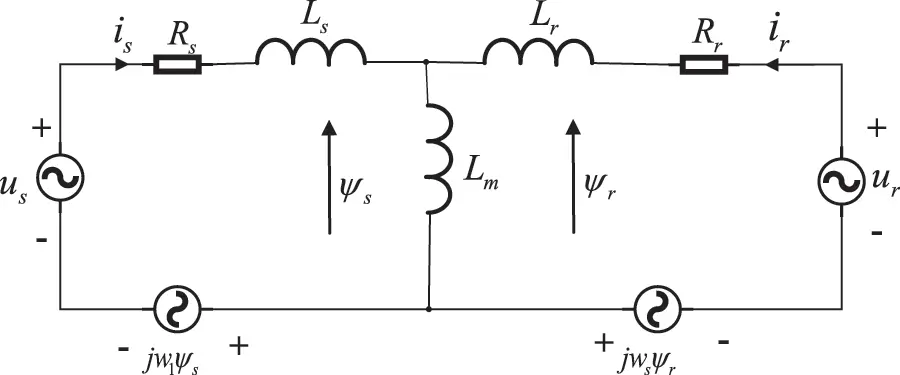Figure 1: T-type equivalent schematic diagram of DFIG system

When the grid voltage is constant, the doubly-fed induction generator operates in a steady state and the stator flux flux amplitude is constant. According to Eqs. (5) and (6), the stator current vector and rotor flux vector can be deduced as Eqs. (7) and (8), respectively.

ψs=Lmims(5)

ims=LsisLm+ir(6)

is=LmLs(ims+ir)(7)

ψr=Lm2Lsims+σLrir(8)

where Ls and Lr are leakage inductances of stator and rotor, respectively; Lm is the mutual inductance; ims is the excitation current of stator; σ = 1 − L2m/LsLr is the magnetic flux leakage coefficient of the generator.

Substituting Eqs. (7) and (8) into Eq. (3), the voltage vector equation under steady state condition of DFIG can be obtained as

{us=Rsis+Lmdimsdt+jω1ψsur=Rrir+σLrdirdt+Lm2Lsdimsdt+jωsψr(9)

In the steady-state condition, the amplitude, phase and frequency of the grid voltage are all regarded as constant values. In the synchronous rotation coordinate system, they can be regarded as two constant DC components. The stator flux ψs is also regarded as constant, which means dims/dt = 0 is true.

However, when a symmetrical fault occurs in the power grid, the stator voltage and the stator flux are no longer constant, and the stator flux ψs oscillates with power frequency attenuation, which can be regarded as composed of the steady-state component ψsf that changes with the power grid voltage and the transient component ψsn that decays with time.

Assume that a three-phase symmetrical fault occurs in the system at the time, then the grid voltage can be expressed as

us=(1p)Uejωst(10)

After the failure, the stator flux is deduced as

ψs=(1p)Ujωseiωst(11)

According to the stator flux differential equation

dψsdt=usRsLsψs(12)

According to Eqs. (7) and (8), the results of solving the differential equation is

ψs(t)=(1p)Ujωsejωst+pUjωsetτs(13)

where U is the stator voltage amplitude, p is the symmetrical sag depth of the grid voltage, and τs is a time constant.

According to Eq. (13), the stator flux is a gradually attenuating quantity, and the degree and time of attenuation are affected by voltage sag depth p and fault occurrence time t. The deeper the voltage sag depth is, the longer the fault occurrence time and the greater the amplitude of attenuation oscillation are. When the grid voltage drops, the change of stator flux cannot be ignored. Meanwhile, according to Eqs. (3) and (4), the change of stator flux will affect the size of rotor voltage, indicating that the influence of flux flux should be considered when controlling the rotor side.

In case of three-phase symmetrical drop of grid voltage, the change track of stator flux is shown in Fig. 2. During DFIG steady-state operation, the stator flux is a circle with radius of 1 . In case of three-phase symmetrical fault, the circular radius reflecting the stator flux track gradually decreases, and finally reaches a new steady-state and forms a new circular track. According to the stator flux trajectory, it can be concluded that the oscillation amplitude of the stator flux ψs is directly proportional to the amplitude p of the symmetrical drop of the grid voltage. The deeper the voltage drop, the greater the oscillation amplitude of the stator flux and the smaller the radius of the circle after reaching stability.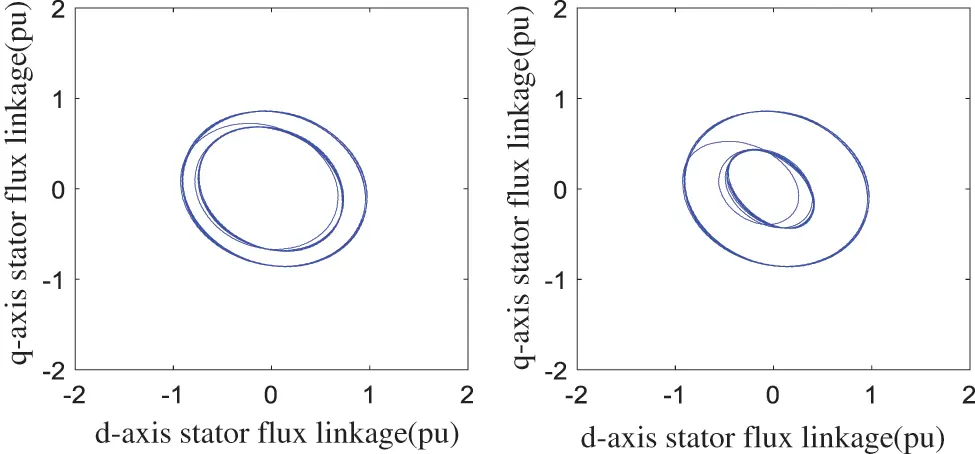Figure 2: Stator flux trajectories with voltage drops of 20% and 50%

2.2 Improved Rotor Side Control Strategy under Symmetrical Faults

In case of grid voltage sag, us and ψs in Eq. (9) no longer remain constant. The simplified DFIG model is usually adopted in traditional control, but is not applicable in dynamic process. According to the stator voltage equation in Eq. (9), we can obtain

dimsdt=1Lm(usRsisjω1ψs)(14)

The rotor side adopts grid voltage orient vector control, namely usd = |us|, usq = 0. By substituting Eq. (14) into Eq. (9), the rotor voltage equation at fault can be obtained as

{urd=Rrird+σLrdirddtωsψrq+LmLs(usdRsisd+ω1ψsq)urq=Rrirq+σLrdirqdt+ωsψrd+LmLs(Rsisqω1ψsd)(15)

where ψsd and ψsq are the stator d-axis and q-axis flux chains; ψrd and ψrq are the rotor d-axis and q-axis flux chains; usd and usq are the stator d-axis and q-axis voltages; urd and urq are the rotor d-axis and q-axis voltages.

Compared with the traditional rotor-side control, the improved control strategy adds the dynamic compensation term. When the grid voltage changes dynamically, the rotor-side control voltage will adjust in real time with the stator voltage change, so as to achieve accurate control. After considering the dynamic process of stator excitation current and adding dynamic compensation term, in order to further improve the low voltage crossing ability of the system, when symmetrical faults occur, the reactive power regulation ability of the converter is given full play to provide reactive power support for the parallel point in the voltage sag period to improve the voltage level of the parallel point.

Since the oscillation amplitude of stator flux ψs is proportional to the degree of network voltage sag, in order to reduce the oscillation degree of ψs, the reactive power should be given priority in the control of rotor side during symmetrical fault. Firstly, the reactive power capacity of the system is calculated according to the degree of grid voltage sags p, and the control reference value of reactive power Qref is obtained, so as to maintain the relative stability of grid-connected voltage. Then, the active power is sent out as far as possible according to the limit of converter transmission power, so as to realize the transmission of system power.

The rotor side adopts stator voltage directional control, and the d-axis and q-axis components of stator current are calculated as follows:

{isd=LmLsirdisq=LmLs(Usω1Lm+irq)(16)

According to the system transmission power formula, the rotor current can be obtained as

{ird=2PsLs3usLmirq=usLmw1LsisqLm(17)

where isd and isq are the stator d-axis and q-axis currents; ird and irq are the rotor d-axis and q-axis currents.

Since Eq. (18) is valid, Eq. (19) can represent the limit value of stator reactive power transmission.

ir=ird2+irq2(18)

isqmax=(LmLsirmax)249(Psus)2usω1Ls(19)

where Ps is the active power transmitted by the system, and isqmax is the maximum rotor current.

As shown in Fig. 3 is the improvement of the rotor side control strategy structure. Compared with the traditional rotor lateral control, the improved control strategy considers the fault during the dynamic process of stator current excitation, and changes the traditional control of dynamic compensation amount, as shown in Fig. 3 shadow part. At the same time, during the fault according to the depth of the grid voltage drop of the dynamic change of reactive power reference value, it provides reactive power to support the system, accelerate voltage recovery and improve low voltage pass levels.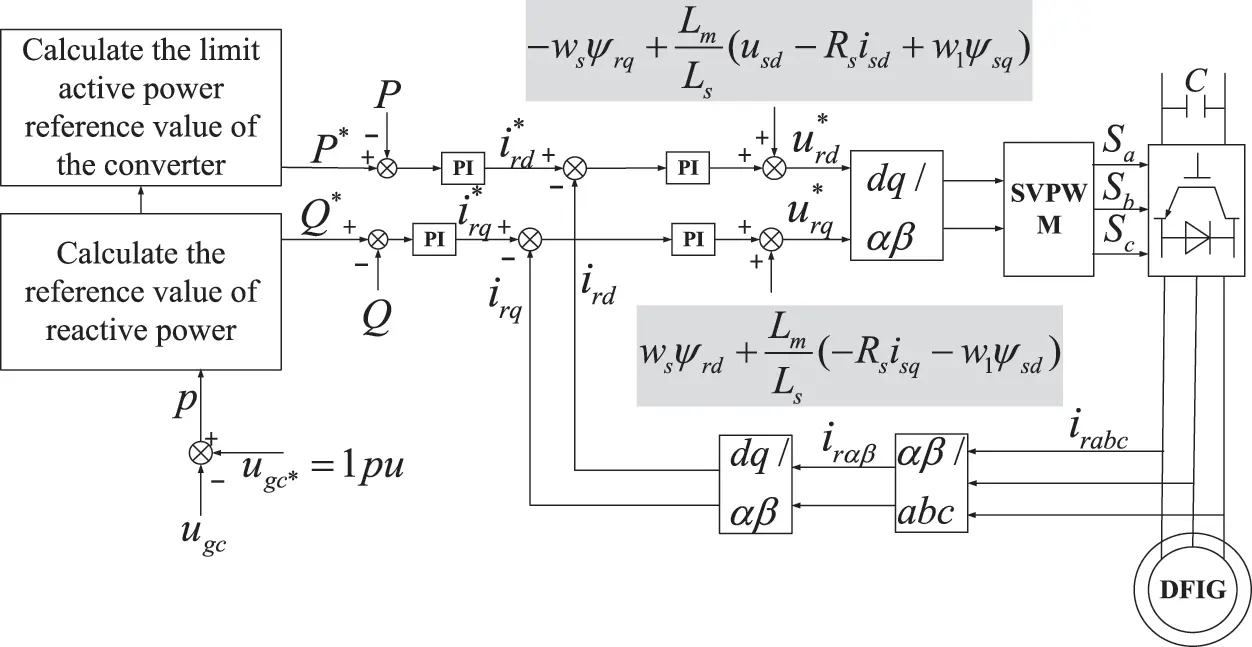Figure 3: Structure diagram of improved rotor side control strategy under symmetrical faults

After the reference value of reactive power is determined, the reference value of active power is adjusted according to the transmission capacity limit of the converter, and the active power is transmitted to the power grid.

3  Operation Characteristics of DFIG under Asymmetrical Faults and Improved Rotor Side Control Strategy

3.1 Operation Characteristics of DFIG under Asymmetrical Faults

When the grid voltage drops asymmetrically, negative sequence components will appear on the stator side of DFIG . According to Eq. (1), the voltage equation of DFIG in the synchronous rotation coordinate system can be deduced as

{usdq+=Rsisdq++dψsdq+dt+jω1ψsdq+urdq+=Rrirdq++dψrdq+dt+jωslip+ψrdq+usdq=Rsisdq+dψsdqdt+jω1ψsdqurdq=Rrirdq+dψrdqdt+jωslipψrdq(20)

where usdq+ and urdq+ are the positive sequence voltage vectors of stator and rotor; usdq and urdq are the negative sequence voltage vectors of stator and rotor; ωslip+ and ωslip− are the positive and negative rotational slips difference frequency, respectively.

{ωslip+=ωr+ω1ωslip=ωrω1(21)

According to Eqs. (2) and (21), the flux linkage formula in positive and negative order can be deduced as follows:

{ψsdq+=Lsisdq++Lmirdq+ψrdq+=Lrirdq++Lmisdq+ψsdq=Lsisdq+Lmirdqψrdq=Lrirdq+Lmisdq(22)

where ψsdq+ and ψrdq+ is the positive sequence flux vectors of stator and rotor; ψsdq and ψrdq is the negative sequence flux vectors of stator and rotor.

After positive and negative sequence decomposition of stator voltage and flux linkage, positive sequence active power Ps+ and reactive power Qs+ absorbed from the grid can be expressed as

{Ps+=32(usd+isd++usq+isq+)Qs+=32(usq+isd+usd+isq+)(23)

Since stator voltage vector control is adopted at the rotor side, and the q-axis voltage is 0: usq = 0. Then the positive sequence active power and reactive power components can be expressed as

{Ps+=32usd+isd+Qs+=32usd+isq+(24)

3.2 Improved Rotor Side Control Strategy under Asymmetrical Faults

In the case of asymmetrical faults, over-current and over-voltage will appear at the rotor side. In addition to the positive sequence component, the negative sequence component with double frequency also exists in the rotor side. Since the traditional control strategy cannot control the negative sequence components alone, the proposed rotor-side control strategy under asymmetrical faults first decomposes the system voltage, current and flux equations into positive and negative sequence decomposition, and then controls them in the positive and negative sequence rotation coordinate system. In order to reduce the over-current phenomenon at the rotor side, the improved rotor-side control is used to set and suppress the reference value of the positive sequence component irdq+ and negative sequence component irdq of the rotor current under fault conditions.

Ignoring the reluctance and stator transient process, the relationship between the positive-sequence rotor current and stator current can be deduced according to Eqs. (20) and (22).

{isd+=LmLsird+isq+=usd+ω1LsLmLsirq+(25)

where isd+ and isq+ are the positive sequence d-axis and q-axis components of stator current, and ird+ and irq+ are the positive sequence d-axis and q-axis components of rotor current.

In order to suppress the positive sequence component of rotor current and ensure that the reactive power output by the system is 0, isq+ = 0. The reference value of the positive sequence component of rotor current can be set as

{ird+=0irq+=usd+ω1Lm(26)

Similar to the positive sequence current, the relationship between the negative sequence rotor current and the stator current can be deduced according to Eqs. (20) and (22) as follows:

{ird=LsLmisdirq=usdω1LmLsLmisq(27)

In the process of setting positive and negative sequence current in the rotor reference value, with the increase of voltage unbalance degree, the setting of the positive sequence voltage and negative sequence voltage after coordinate transformation to get the total voltage reference may be exceeding the maximum voltage of the inverter output. The positive sequence is determined after the rotor current reference, also need to consider the inverter output voltage limit.

The reference values of the current and voltage components of the negative-sequence rotor are set according to the limit value of the output voltage. The positive and negative sequence equation of the rotor voltage can be expressed as

{urd+=ωslip+(Lmω1Lsusd++σLrirq+)urq+=ωslip+(Lmω1Lsusq++σLrird+)urd=ωslip(Lmω1Lsusd+σLrirq)urq=ωslip(Lmω1Lsusq+σLrird)(28)

In order to minimize the rotor negative sequence current component and suppress the rotor over-current phenomenon, the reference value of rotor negative sequence voltage can be set as

{urd=ωslipLmω1Lsusdurq=ωslipLmω1Lsusq(29)

Considering the output voltage limit of the converter, the rotor voltage can be approximately expressed as

urmax(urd+)2+(urq+)2+(urd)2+(urq)2(30)

In the process of grid voltage sag, the grid-side control strategy keeps the DC side voltage udc unchanged. Due to the step-down characteristics of the converter, the rotor voltage will be limited. The maximum output voltage of the rotor side is closely related to the DC side voltage level, which can be expressed as

urmax=kmaxudc(31)

The value of the constant kmax is affected by the modulation mode. In the SVPWM modulation mode, kmax =√3/3 . In the process of grid voltage sag, rotor converter (RSC) has transmission limit. If it is not restricted, when the grid voltage imbalance is large, the target value of rotor voltage will be larger than the transmission voltage limit if it is directly controlled according to the reference value given in Eq. (29). Due to the RSC transmission voltage limit, the negative sequence voltage of the rotor cannot be effectively controlled. When the grid-side converter is controlled, the DC side voltage is controlled at about 1200 V. According to Eq. (31), the transmission limit of RSC can be calculated to be about 692 V.

On the premise that the positive sequence rotor current control reference value is given, the negative sequence voltage limit that can be transmitted can be calculated according to Eq. (28), and the negative sequence voltage reference value can be limited. When the control requirements are met and the sum of positive and negative sequence voltages is within the RSC voltage transmission limit, the structure of rotor-side control strategy in the case of asymmetrical grid voltage fault is shown in Fig. 4.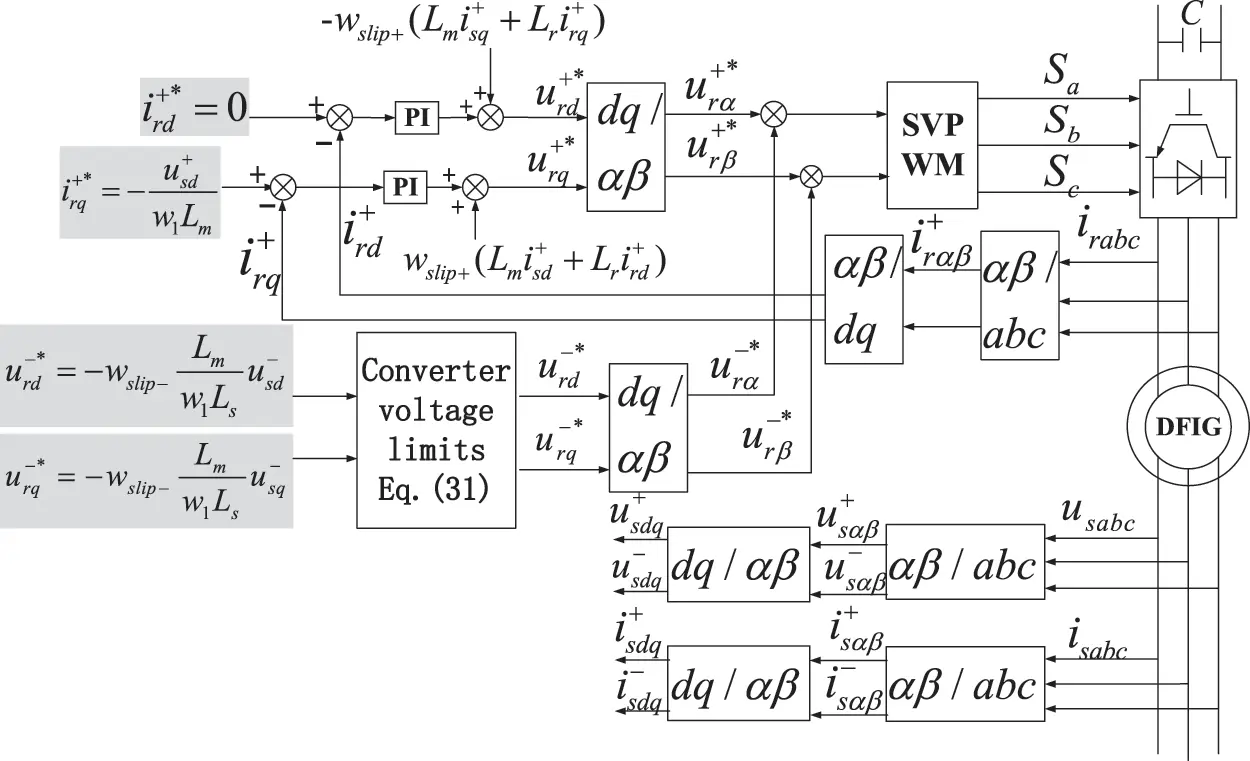Figure 4: Structure diagram of improved rotor side control strategy under asymmetrical faults

4  Fault Type Detection and Rapid Control

Since the occurrence of grid faults is stochastic in time and uncertain in type, the improved rotor-side control strategy for symmetrical faults and asymmetrical faults proposed in this paper can dynamically change according to the actual grid faults and the depth of grid voltage sag to cope with different operating conditions of the grid.

In order to realize the rapid switching of fault type detection and control mode, a grid voltage amplitude detection module is designed at the parallel node. The switching of rotor side control mode is controlled by the positive and negative level signals output by the fault detection module. The control flow chart is shown in Fig. 5. At the beginning, the system operates stably and the grid voltage amplitudes remain constant. The change of power grid voltage amplitude is detected in real time by fault detection module. If the three-phase amplitude of grid voltage is equal and the magnitude remains above 0.9 pu, the system is considered to be in steady-state operation. If it is detected that the three-phase amplitude of grid voltage drops at the same time and the amplitude decreases below 0.9 pu, the control mode fast switching module switches the control mode on the rotor side to the control mode based on symmetrical fault. At the same time, the fault detection module calculates the symmetrical drop depth of power grid voltage, calculates the reference value of reactive power according to the drop depth, calculates the reference value of active power according to Eq. (15), and runs the control block diagram shown in Fig. 3 to realize the switching of rotor side control mode in case of symmetrical fault of power grid. If it is detected that the three-phase amplitude of the grid voltage decreases asymmetrically and the amplitude decreases below 0.9 pu, the control mode fast switching module switches the rotor side control to the control mode under asymmetric fault, and the rotor side control adopts the control method in Fig. 4.Figure 5: Flowchart of fault detection and control switching

5  Simulation Study

5.1 Parameters of Simulation Model

A doubly-fed wind power generation system model was established in Matlab/Simulink to verify the effectiveness and correctness of the improved rotor side control during the symmetrical and asymmetrical faults of the power grid. The specific parameters of DFIG are shown in Table 1.The other parameters of the system are as follows. The reference value of DC side voltage is 1200 V; the voltage of parallel point is 690 V; the frequency is 50 Hz and the DC side capacitance is 0.02 F.

According to the generator datas in Table 1, a simulation system based on 2 MW DFIG as shown in Fig. 6 is established in Matlab/Simullink. In the established simulation model, the parallel node voltage of DFIG is 690 V, and the wind turbine is connected with 35 kV bus through a step-up transformer.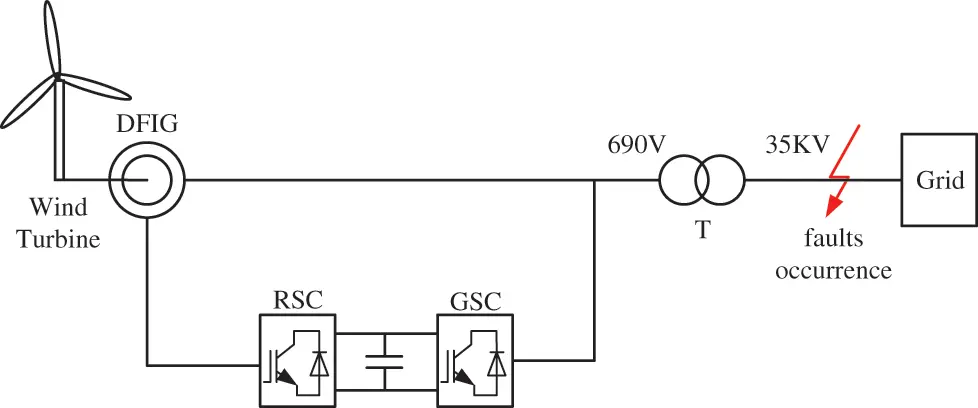Figure 6: Simulation model structure diagram of a 2-MW DFIG system

As shown in the red part of Fig. 6, all the simulation results below are based on the fault occurring on the 35kV bus. The effectiveness of the proposed control strategy is verified by simulating three-phase voltage drop and single-phase voltage drop at this point.

5.2 Simulation Results under Symmetrical Faults

In order to verify the feasibility and superiority of improving the rotor side control under symmetrical fault, according to the national requirements for low voltage ride through, the system can maintain grid connected operation for 0.625 s in case of fault. The system operates stably at the initial stage, and the grid voltage drops by 50% and 80% respectively in three phases at 1s. In order to verify the control performance under long-term fault, the fault is removed in 1.625 s, and the system returns to steady-state operation.

Fig. 7a shows the simulation results of the system when the grid voltage drops symmetrically to 50%. It can be seen from the figure that the grid voltage usabc drops from the original 1 pu to 0.5 pu. During the fault, the DC side voltage udc can be maintained at about 1200 V, which effectively inhibits the DC side voltage rise during the grid voltage drop. At the same time, the amplitude of stator current isabc and rotor current irabc can be kept at 1 pu during the fault, which means there is no rotor over-current phenomenon. According to the waveforms of active power and reactive power, it can be found that the active power of 1 pu is output to the grid side of the system during the steady-state operation, and the value of reactive power remains at 0, and the system operates in the unit power factor state. During the fault period, according to the drop depth p, the system provides a certain amount of reactive power support to the grid to accelerate the voltage recovery. At the same time, due to the limitation of the output power capacity of the converter, the active power transmitted by the system to the grid will also be affected and reduced.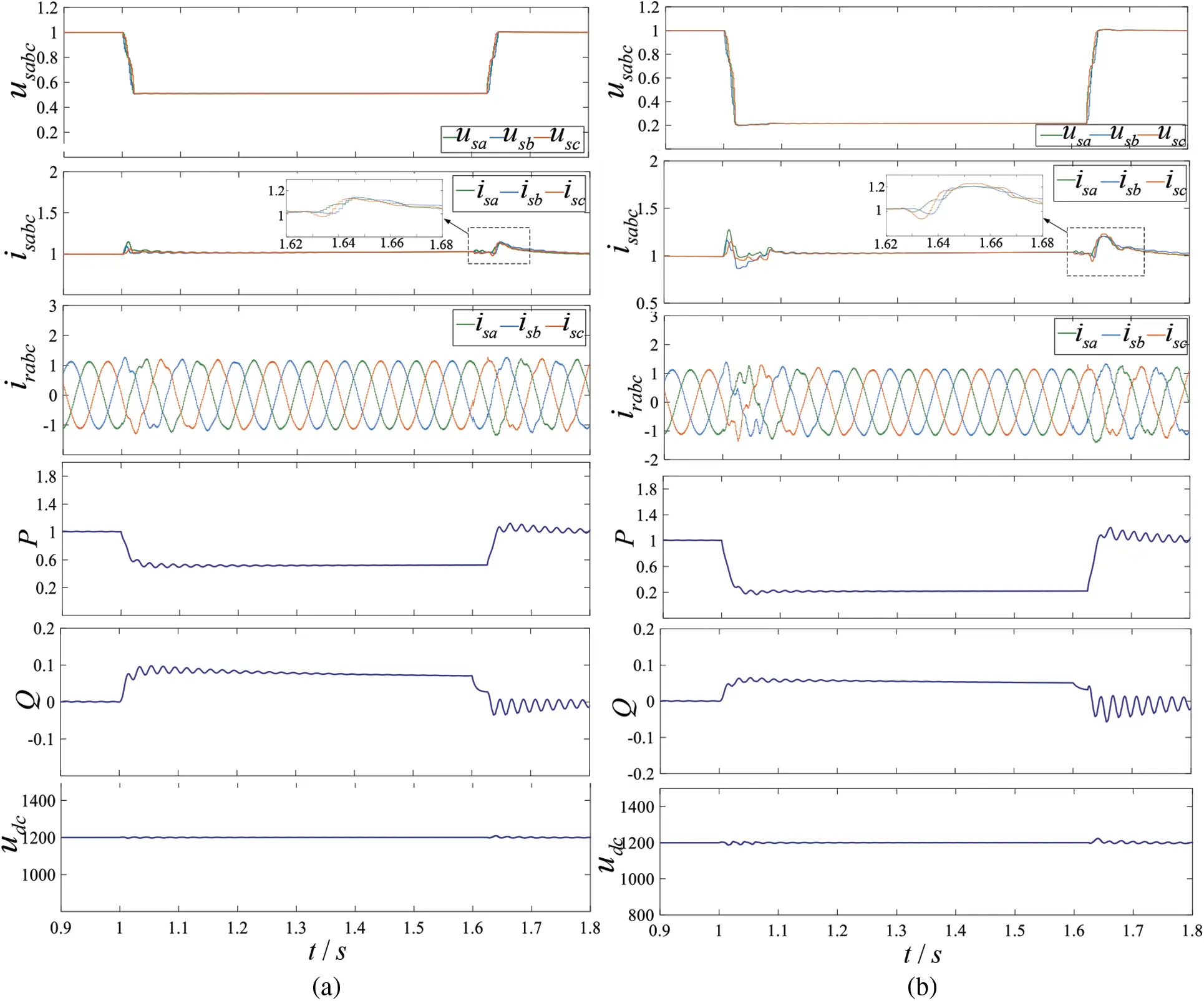Figure 7: Waveforms of grid voltage, stator current, rotor current, grid-side power and DC side voltage with 50% (a) and 80% (b) symmetrical drops of grid voltage

Fig. 7b shows the simulation results of grid voltage, stator current, rotor current, DC side voltage, active power and reactive power when the grid voltage drops 80% symmetrically. Compared with Fig. 7a, the fluctuation of rotor current and DC side voltage increases relatively, especially at the moment of fault occurrence and fault removal, However, the phenomenon of rotor over-current and DC side voltage rise can still be well suppressed.

5.3 Simulation Results under Asymmetrical Faults

Similar to the symmetrical fault simulation, the DFIG system operates in steady state at the beginning, and the asymmetrical fault represented by single-phase voltage sag occurs at 1 s. The fault lasts for 0.625 s, and then the grid voltage returns to normal, and the system operates in steady state. In order to verify the feasibility of rotor side control under long-term asymmetrical fault, the simulation platform is built to test the conditions of high drop degree, namely single-phase voltage drop 50% and 80%.

Fig. 8a shows the simulation results of 50% asymmetric voltage drop of power grid. According to the waveform of power grid voltage usabc, it can be seen that the system has an asymmetrical fault of 50% phase A voltage drop in 1 s.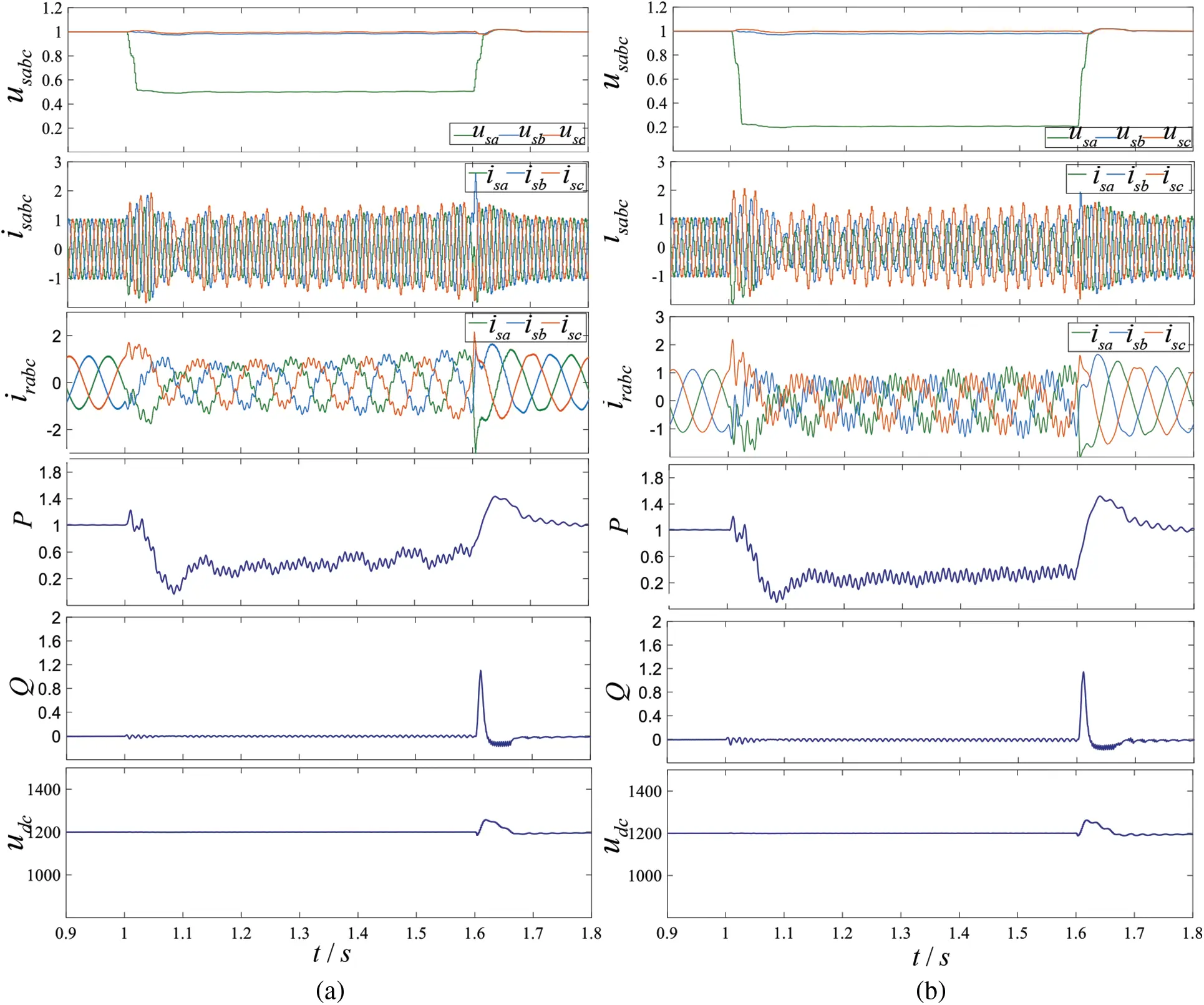Figure 8: Waveforms of grid voltage, stator current, rotor current, grid-side power and DC side voltage with 50% (a) and 80% (b) asymmetrical drops of grid voltage

During the fault period, due to the influence of negative sequence component and transient process, the stator current and rotor current have certain distortion, and the increase of rotor current is restrained. At the same time, the DC side voltage can keep stable during the fault and fluctuate at the time of fault clearing, which effectively reduces the DC side voltage during the fault. For the grid side output power, the unit power factor operation is maintained in steady state, the output active power Ps is 1 pu, and the reactive power Qs is 0. During the fault period, due to the grid voltage drop, the rotor side control strategy is switched from steady-state control to asymmetrical fault control, the active power will be reduced correspondingly, and the reactive power can still fluctuate around 0.

The improved rotor control strategy for asymmetrical fault requires the system to operate normally when the sag is high. Fig. 8b shows the simulation results of asymmetrical fault with 80% phase A sag of grid voltage. Under the condition of deep sag (p = 0.8), the fluctuation degree of rotor current and stator current increases, and the variation of DC side voltage also increases to a certain extent. The active power is stable at about 0.2 times of the steady-state operation, and the reactive power fluctuates around 0 during the fault. By comparing with the result chart of single-phase voltage drop of 50%, it is found that the higher the degree of single-phase voltage drop, the greater the fluctuation of rotor current, stator current and DC side voltage, and the control performance will also be affected.

5.4 Simulation Results of Coordinated Control for Symmetrical and Asymmetrical Faults

The fault detection and control mode fast switching module are added in the system, which can deal with all kinds of grid voltage dips. In the actual operation condition, the occurrence of fault is not controlled by human. In order to verify the real-time and correctness of the designed fault detection and control mode fast switching module, the occurrence and switching of symmetrical fault and asymmetrical fault are comprehensively tested in the simulation platform, that is, the initial steady-state operation of the system, the single-phase voltage drop 50% of the asymmetrical fault occurs in 1 s, and the fault intensifies in 1.3 s, and evolves into the symmetrical fault of three-phase voltage drop 50%, At 1.625 s, the fault is removed and the grid voltage returns to the steady value.

The simulation results are shown in Fig. 9. At the moment of single-phase fault, the rotor side control is switched to asymmetrical fault control. During the asymmetrical fault period, the rotor over-current phenomenon is suppressed, and the active power is reduced within the allowable range. At 1.3 s, according to the grid voltage usabc, it can be seen that the grid voltage has three-phase symmetrical drop, the control mode is switched to symmetrical fault control, the rotor current and stator current have a decreasing trend, and it can provide certain reactive power support for the grid. During symmetrical fault and asymmetrical fault, the DC side voltage can be maintained at about 1200 V and the fluctuation amplitude is reduced.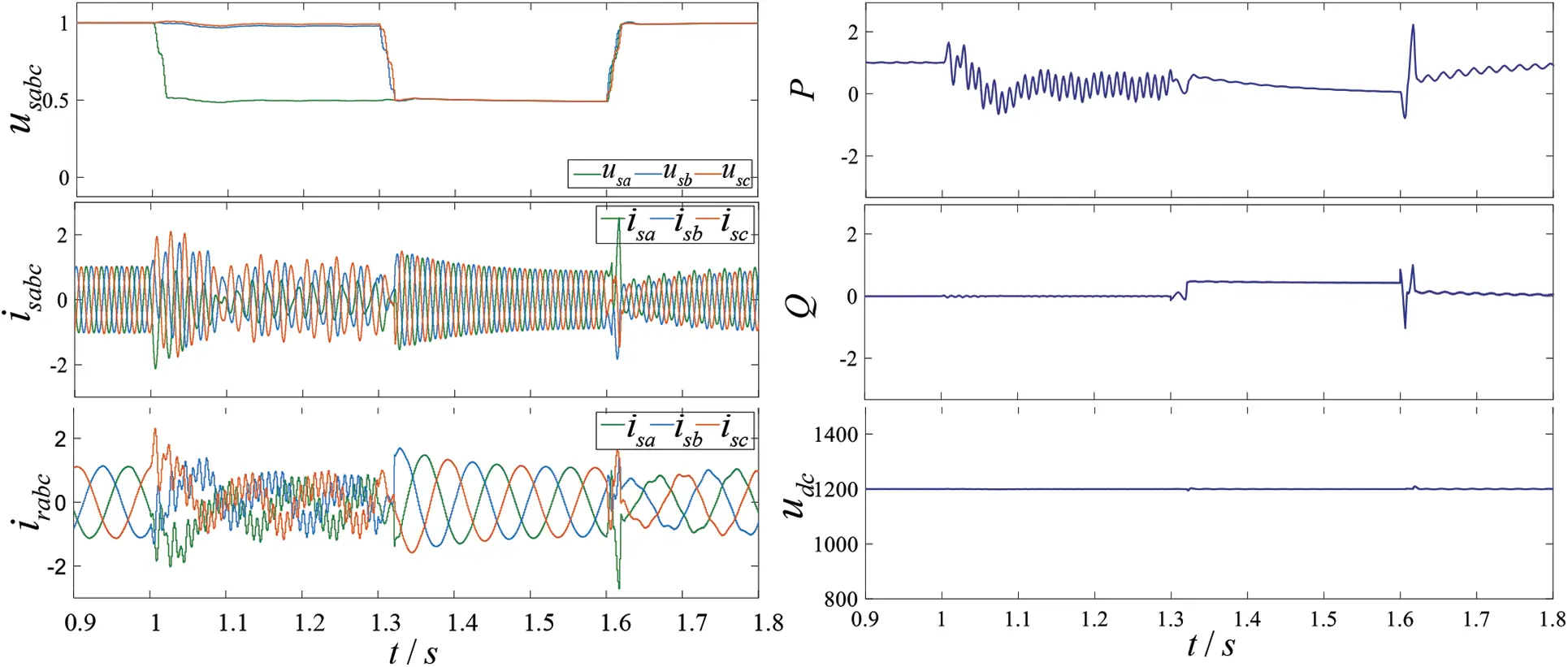Figure 9: Waveforms of grid voltage, stator current, rotor current, grid-side power and DC side voltage with 50% symmetrical and asymmetrical sag of grid voltage

According to the simulation results in Fig. 9, it also can be seen that the system can quickly switch the rotor side control mode according to the fault detection results. At 1, 1.3 and 1.625 s, the rotor side control switches to the rotor side control strategy under asymmetrical fault, the rotor side control strategy under symmetrical fault and the rotor side control strategy under steady state respectively. On the premise that symmetrical fault control strategy and asymmetrical fault control strategy are effective, respectively, the system can coordinate different rotor side control methods to deal with different types of faults and complex operating conditions. The proposed control strategy is not two separate parts. The two control modes can be combined through fault detection and control mode switching module.

5.5 Comparative Analysis Results

In order to verify the effectiveness and novelty of the proposed control strategy, Fig. 10 shows the comparison between the traditional control strategy and the improved control strategy in case of symmetrical fault in the power grid. According to Fig. 10a, it can be seen that the grid voltage has a drop depth of 50% at 1 s and the steady-state operation is restored at 1.625 s. Figs. 10b and 10c show the waveforms of rotor current and stator current of traditional control strategy and improved control strategy in case of symmetry fault, respectively. When the system adopts traditional control, the rotor current and stator current will increase to about twice the original during fault, which endangers the safety of the system. The improved control strategy can keep the amplitude of rotor current and stator current at 1 pu during fault, and can effectively suppress the overcurrent phenomenon. Fig. 10d shows the voltage waveform at the DC side of the system. Compared with the traditional control strategy, the improved control strategy can effectively suppress the voltage fluctuation and improve the stability of the system.Figure 10: Comparison waveforms between improved rotor control strategy and traditional rotor control strategy under symmetrical fault

Fig. 11 shows the comparison waveform between the traditional control strategy and the improved control strategy in case of asymmetrical fault represented by 50% of phase A voltage drop in the power grid. Compared with Figs. 11c and 11d, it can be found that the improved rotor control strategy also has a good suppression effect on the rotor current under asymmetrical fault. For the DC voltage waveform shown in Fig. 11b, the improved control strategy can reduce the DC voltage fluctuation during the fault, and there will be a short voltage rise of about 40 V at 1.625 s, which is acceptable to the system.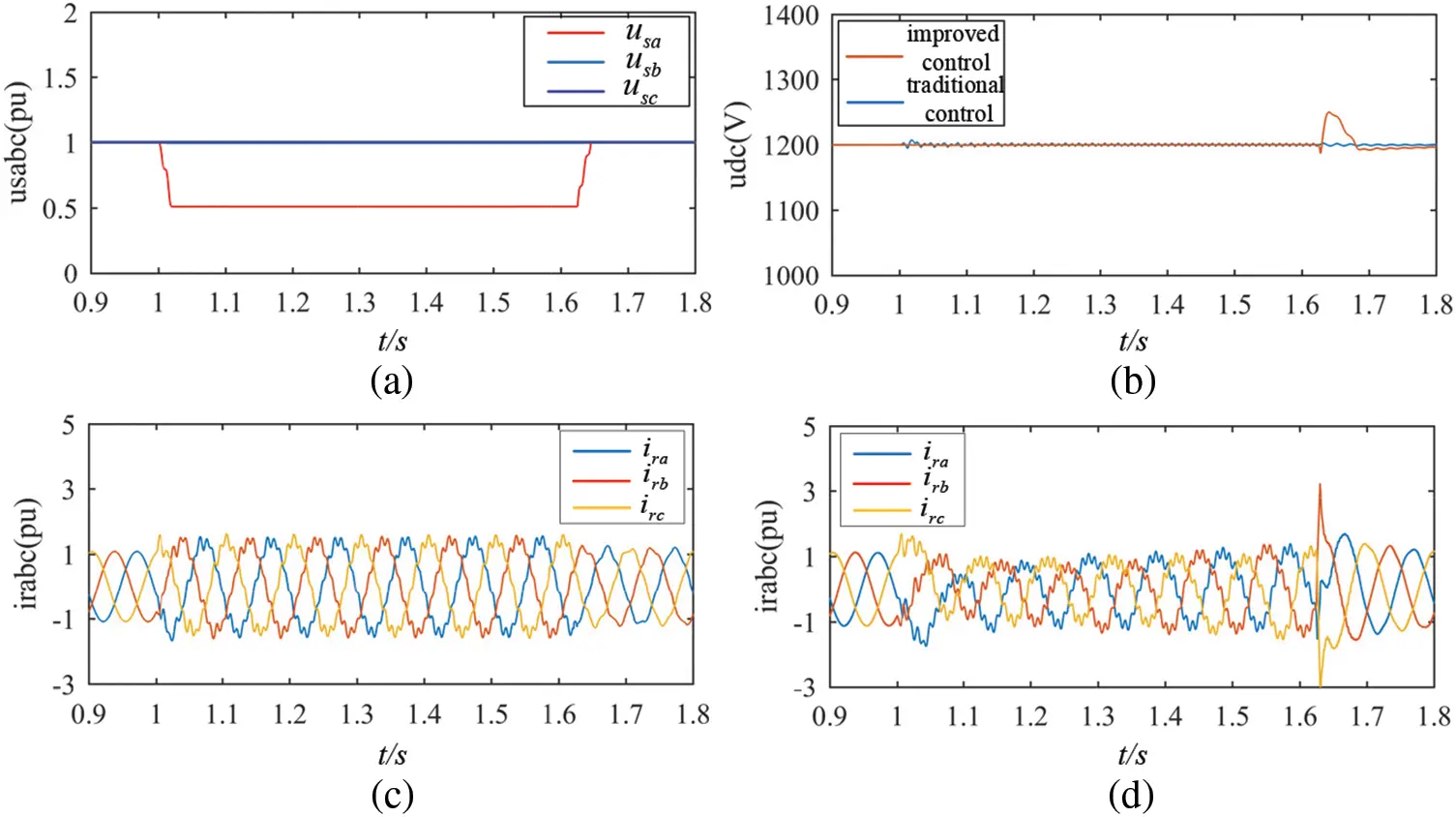Figure 11: Comparison waveforms between improved rotor control strategy and traditional rotor control strategy under asymmetrical fault

The control strategy proposed in this paper comprehensively considers various fault types of power grid. Combined with symmetrical fault and asymmetric fault, it can deal with different faults of power grid at the same time. It has a good inhibitory effect on the rise of DC voltage during low voltage ride through, and compared with the general control mode, it can reduce the voltage fluctuation during fault. At the same time, in order to enhance the flexible conversion of control strategy, the fault type detection and control mode switching module can switch the control mode in time according to the fault type and control the system dynamically.

6  Conclusions

In this paper, because of the phenomenon of symmetrical and asymmetrical faults on the grid side of DFIG wind power system, an improved rotor side control strategy based on doubly fed wind turbine is designed, which can deal with different types of grid faults and voltage drop. At the same time, in order to adapt to the complex operation conditions in actual operation, a fault detection and fast switching module of rotor side control mode is also designed. The conclusions of this paper are as follows:

1.    When three-phase symmetry and three-phase asymmetry voltage sags occur in the power grid, represented by 20%, 50% and 80% voltage sags in the simulation, the improved rotor side control mode can well inhibit the rotor overcurrent phenomenon and the rise of DC bus voltage (maintained at 1200 V). Meanwhile, it can improve the safety and stability of the system and the low-voltage ride through level.

2.    The designed fault detection and rotor side control mode fast switching module can make the symmetrical faults and asymmetrical faults control mode switch correctly when the power grid fault type changes. In the simulation, the feasibility of switching is simulated by steady-state operation and alternating operation of symmetrical fault and asymmetric fault with different degrees of voltage drop. It can dynamically track the operation mode of power grid, and has good flexibility and adaptability.

Acknowledgement: Sincere thanks are due to professor Ying Zhu of Hohai University for providing lots of constructive suggestions.

Funding Statement: The authors highly acknowledge the technology financial assistance provided by Jiangsu Frontier Electric Technology Co., Ltd. (KJ202003).

Conflicts of Interest: The authors declare that they have no conflicts of interest to report regarding the present study.

## References

1. Jia, J. C., Liu, J., & Zhang, Y. G. (2011). Research on dynamic characteristics of stator flux of doubly-fed induction generator under power grid voltage fault. Proceedings of the CSEE, 31(3), 90-96. [Google Scholar]
2. Lin, D. M., Sun, Y. Y., & Zhang, X. L. (2011). Transient characteristics and reactive power coordinated control of DFIG under voltage asymmetrical sudden rise. Electric Drive, 51(4), 34-39. [Google Scholar]
3. Garibay, A. J., Resendiz, J. R. J., & Correa, J. J. (2018). Analysis of rotor current impact on DFIG-WECS under fault condition. IEEE Latin America Transactions, 16(2), 329-334. [Google Scholar] [CrossRef]
4. Zhu, Y., Sha, J., & Zhao, J. (2020). Simulation of low voltage ride capability of wind farm with different units. Power Generation Technology, 41(3), 328-333. [Google Scholar]
5. Liu, T. Y., Zhang, Y. H., Wang, M., Zhang, Y. T., Qin, X. H. (2021). Short-circuit current calculation method of double-fed wind turbine considering control strategy switching process. Proceedings of the CSEE, 41(10), 3330–3338+3659.
6. Zhang, H., Zhang, L. Z., & Tao, Y. Y. (2013). Research on electromagnetic transient process and rotor side control strategy of DFIG under grid voltage sags. Hydropower and New Energy, 3, 68-73. [Google Scholar]
7. Wang, G. X., & Si, X. F. (2017). Joint control strategy for low voltage crossing in doubly-fed wind power system. Electric Drive, 47(11), 50-54. [Google Scholar]
8. Liu, J. (2014). Research on control strategy of double-fed wind power generation system (Ph.D. Dissertation). North China Electric Power University.
9. Cao, L. N. (2021). Key technology of low voltage crossing in doubly-fed wind power generation system. Communications Power Sources Technology, 38(1), 229-231, 234. [Google Scholar]
10. Fsaha, M. G., Baseem, K., & Hassan, H. A. (2020). Analyzing low voltage ride through capability of doubly fed induction generator based on wind turbine. Computers & Electrical Engineering, 86, [Google Scholar]
11. Yang, R. H., & Jin, J. X. (2021). Unified power quality conditioner with advanced dual control for performance improvement of DFIG-based wind farm. IEEE Transactions on Sustainable Energy, 12(1), 116-126. [Google Scholar] [CrossRef]
12. Zou, Z., Xiao, X., & Liu, Y. (2016). Integrated protection of DFIG-based wind turbine with a resistive-type SFCL under symmetrical and asymmetrical faults. IEEE Transactions on Applied Superconductivity, 26(7), 1-5. [Google Scholar] [CrossRef]
13. Xiao, X., Yang, R., & Chen, X. (2018). Integrated DFIG protection with a modified SMES-FCL under symmetrical and asymmetrical faults. IEEE Transactions on Applied Superconductivity, 28(4), 1-6. [Google Scholar] [CrossRef]
14. Chen, J. M., Wang, Y. H., & Zhu, M. X. (2019). Improved rotor braking protection circuit and self-adaptive control for DFIG during grid fault. Energies, 12(10), 1994. [Google Scholar] [CrossRef]
15. Xin, B. L. (2019). Protection scheme simulation of low voltage through DC side unload circuit in double-fed wind power generation system. Electronic Technology and Software Engineering, 21, 197-198. [Google Scholar]
16. Wang, Y. D., Su, J. H., & Wang, H. N. (2021). Brushless doubly fed generator fault ride-through strategy. Journal of motor and control, 25(2), 28-36. [Google Scholar]
17. Li, X., Zhang, X., & Lin, Z. (2018). An improved flux magnitude and angle control with LVRT capability for DFIGs. IEEE Transactions on Power Systems, 33(4), 3845-3853. [Google Scholar] [CrossRef]
18. Saeed, M. A., Hafiz, M. K., & Arslan, A. (2018). Analyzing effectiveness of LVRT techniques for DFIG wind turbine system and implementation of hybrid combination with control schemes. Renewable and Sustainable Energy Reviews, 81, [Google Scholar]
19. Basak, R., Bhuvaneswari, G., & Pillai, R. R. (2020). Low-voltage ride-through of a synchronous generator-based variable speed grid-interfaced wind energy conversion system. IEEE Transactions on Industry Applications, 56(1), 752-762. [Google Scholar] [CrossRef]
20. Li, S., Tong, L., & Liu, S. X. (2020). Control Strategy of photovoltaic grid-connected inverter under unbalanced grid voltage. Journal of Chongqing University of Technology (Nature Science), 34(9), 259-266. [Google Scholar]
21. Yang, S. Y., Chen, L. W., & Sun, D. Y. (2014). Low voltage crossing transient control strategy of doubly-fed wind turbine under asymmetrical power grid fault. Automation of Electric Power Systems, 38(18), 13-19. [Google Scholar]
22. Hiremath, R., Moger, T. (2020). Comparison of LVRT enhancement for DFIG-based wind turbine generator with rotor-side control strategy. 2020 International Conference on Electrical and Electronics Engineering (ICE3), pp. 216–220. Antalya, Turkey.
23. Yin, G. L., Wang, H. L., & Wang, S. (2020). Improvement of vector control strategy for doubly-fed wind motor under grid voltage sag. Journal of Power Systems and Automation, 32(10), 111-116. [Google Scholar]
24. Geng, H., Liu, C., & Yang, G. (2013). LVRT capability of DFIG-based WECS under asymmetrical grid fault condition. IEEE Transactions on Industrial Electronics, 60(6), 2495-2509. [Google Scholar] [CrossRef]
25. Chen, L., Zhang, B., & Fan, X. (2020). Asymmetrical fault ride-through control strategy for rotor-side converter of DFIG. IEEE Transactions on Energy Conversion, 35(2), 1046-1053. [Google Scholar] [CrossRef]
26. Christiansen, W., Johnsen, D. R. (2006). Analysis of requirements in selected grid codes. Orsted-DTU Section of Electric Power Engineering, Technical University of Denmark (DTU).
27. Tian, J. F. (2020). Research on operation control of doubly fed wind turbine under unbalanced grid voltage (MA Thesis). Lanzhou University of Technology, China.
28. Wang, M., Shi, Y., Zhang, Z., Shen, M., & Lu, Y. (2017). Synchronous flux weakening control with flux linkage prediction for doubly-fed wind power generation systems. IEEE Access, 5, 5463-5470. [Google Scholar] [CrossRef]
29. Xu, H., Zhang, Y., Li, Z., Zhao, R., & Hu, J. (2020). Reactive current constraints and coordinated control of DFIG’s RSC and GSC during asymmetric grid condition. IEEE Access, 8, 184339-184349. [Google Scholar] [CrossRef]
30. Xiao, S., Geng, H., & Zhou, H. (2013). Analysis of the control limit for rotor-side converter of doubly-fed induction generator-based wind energy conversion system under various voltage dips. IET Renewable Power Generation, 7(1), 71-81. [Google Scholar] [CrossRef]

Yan, Q., Yuan, C., Gu, W., Liu, Y., Tang, Y. (2023). Coordinated Rotor-Side Control Strategy for Doubly-Fed Wind Turbine under Symmetrical and Asymmetrical Grid Faults. Energy Engineering, 120(1), 49–68.This work is licensed under a Creative Commons Attribution 4.0 International License , which permits unrestricted use, distribution, and reproduction in any medium, provided the original work is properly cited.

View

• ### 352